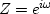Next: The quadrature filter Up: HILBERT TRANSFORM Previous: HILBERT TRANSFORM

## A Z-transform view of Hilbert transformation

Let xt be an even function of t. The definitiongives; so(1) (2)
Now make up a new function Y(Z) by replacing cosine by sine in (2):(3)
Recalling that, we see that all the negative powers of Z cancel from X(Z)+iY(Z), giving a causal C(Z):(4)
Thus, for plot pairs, the causal response is ct, the real part of the FT is equation (2), and the imaginary part not usually shown is given by equation (3).Next: The quadrature filter Up: HILBERT TRANSFORM Previous: HILBERT TRANSFORM
Stanford Exploration Project
10/21/1998